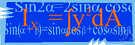# mathematical notation

Also found in: Thesaurus, Wikipedia.
ThesaurusAntonymsRelated WordsSynonymsLegend:
 Noun 1mathematical notation - a notation used by mathematicians  mathematical statement - a statement of a mathematical relationnotation, notational system - a technical system of symbols used to represent special thingsnumber representation system, number system, numeration system, system of numeration - any notation for the representation of numbersbinary notation - any notation that uses 2 characters (usually 0 and 1)decimal notation - any notation that uses 10 different characters (usually the digits 0 to 9)octal notation - any mathematical notation that uses 8 different characters (usually the digits 0 to 7)duodecimal notation - any notation that uses 12 different charactershexadecimal notation, sexadecimal notation - any notation that uses 16 different characterssign - a character indicating a relation between quantities; "don't forget the minus sign"decimal point, percentage point, point - the dot at the left of a decimal fractionexponent, index, power - a mathematical notation indicating the number of times a quantity is multiplied by itselffixed-point notation, fixed-point representation system - a radix numeration system in which the location of the decimal point is fixed by conventionfloating-point notation, floating-point representation system - a radix numeration system in which the location of the decimal point is indicated by an exponent of the radix; in the floating-point representation system, 0.0012 is represented as 0.12-2 where -2 is the exponentinfix notation - a notation for forming mathematical expressions using parentheses and governed by rules of operator precedence; operators are dispersed among the operandsparenthesis-free notation - a notation for forming mathematical expressions that does not use parentheses to delimit components
Based on WordNet 3.0, Farlex clipart collection. © 2003-2012 Princeton University, Farlex Inc.
References in periodicals archive ?
For example, the acronym BODMAS (or BOMDAS, or DAMNUS, or BIMDAS, depending on the instructor's inclinations) exists to help students acculturate to the linguistic structures of modern mathematical notation, which is in some ways arbitrary (Watson, 2010).
In Section 2, we state the terminology and mathematical notation that will be used throughout this paper.
Clever analogies sit alongside equations and mathematical notation, both of which share space with anecdotes from his career.
Their math query language offers an alternative way to describe mathematical expressions that is more consistent and less ambiguous than conventional mathematical notation.
Enlightening Symbols: A Short History of Mathematical Notation and Its Hidden Powers
It uses the schema as a tool, helping the student to elaborate on and translate the information from the word problem into a semantic representation, to plan ways to represent the problem in mathematical notation, and to solve the problem (Montague & Jitendra, 2012).
One of the central achievements of Colyvan's textbook is the way it seamlessly weaves together introductory material on the debate over mathematical realism with state of the art research on mathematical explanation, the applicability of mathematics in science and mathematical notation. The book would therefore serve as a useful touchstone for a graduate student writing a research thesis on one of these interesting topics.
Going through Principia chapter by chapter, he places Newton's writings in their historical context as well as "translating" the book into modern English language and mathematical notation, explicating specific passages, showing derivations of the important equations in exquisite detail and maintaining a level of both entertainment and accessibility as well as mathematical and scientific rigor throughout.
(Surely all of you quants know Leibniz as the creator of infinitesimal calculus and mathematical notation.)
In this paper we focus on the importance of understanding the notion of function, mathematical notation, and properties used in relation to functions in order to work with logarithms.
This, however, is not a reasonable justification, as it is one thing to adopt simplifying assumptions (which seems perfectly reasonable in empirical work) or abuse mathematical notation by representing linear demands when they are not, and another thing to allow negative quantities and ad hoc consumer surplus measures.

Site: Follow: Share:
Open / Close